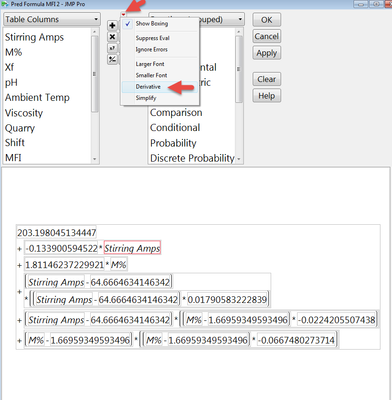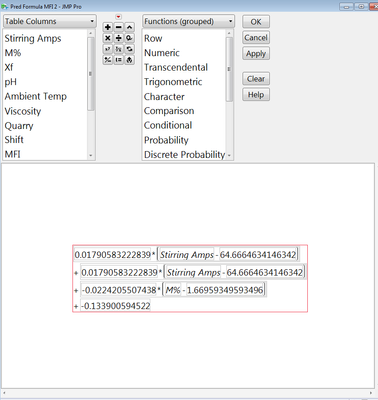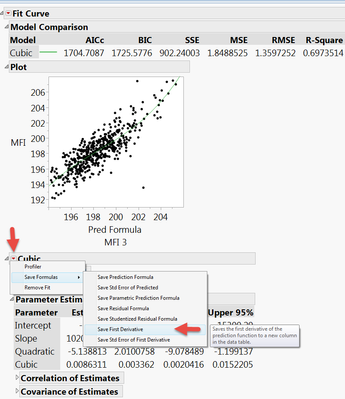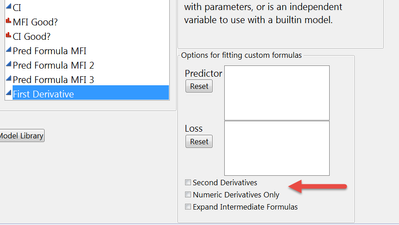Our World Statistics Day conversations have been a great reminder of how much statistics can inform our lives. Do you have an example of how statistics has made a difference in your life? Share your story with the Community!
Choose Language Hide Translation Bar
Highlighted

## Is it possible to solve differential equations in JMP ?

Hello All ,

I would like to know if it is possible to solve differential equations in JMP ? If yes - can you please post an example showing how to do it or provide any blog posts that explain this ? If no - can some one explain why not  ?

Note : I do not wish to invoke Matlab to achieve this .

Best

Uday

Best
Uday
1 ACCEPTED SOLUTION

Accepted Solutions
Highlighted

## Re: Is it possible to solve differential equations in JMP ?

There is a section in the JSL Scripting Guide

Help==>Books==>Scripting Guide

that deals with the calculation of derivatives.  In the JMP 12 Scripting Guide, it starts on page 265.

Jim
3 REPLIES 3
Highlighted

## Re: Is it possible to solve differential equations in JMP ?

There is a section in the JSL Scripting Guide

Help==>Books==>Scripting Guide

that deals with the calculation of derivatives.  In the JMP 12 Scripting Guide, it starts on page 265.

Jim
Highlighted

## Re: Is it possible to solve differential equations in JMP ?

There are at least two ways to calculate a derivative or at least save the formula for the first derivative.

For the first way you need to save a formula or create a formula column.  To take the derivative go to the column of interest, right click on the column header and then click Formula.  When the formula editor opens you will see the formula and you must select which variable you want to take your derivative with respect to.The second way is in the nonlinear platform.  You again need to create a model, but instead of saving the prediction formula you will save the formula for the first derivative.You can also specify that you want second derivatives and/or numeric only derivatives in the nonlinear platform model dialogue.

HTH

Bill

Highlighted

## Re: Is it possible to solve differential equations in JMP ?

JSL has a derivative function.  I've forgotten all my calculus, so I can't explain the last three terms, but I made a small test harness, and it works.

// make a really ugly formula

fx = Expr(

A * x ^ W + B * x ^ Z + C * x + D * Sin( E * x ) + F * x * Log( G * x ) + Tan( x ) * Exp( x )

);

// ask JMP to find the derivative with respect to x.  p for prime.

fxp = Derivative( Name Expr( fx ), x );

W * x ^ (W - 1) * A

+ Z * x ^ (Z - 1) * B

+ C

+ E * Cosine( E * x ) * D

+ F * Log( G * x ) + G / (G * x) * F * x

+ 1 / Cosine( x ) ^ 2 * Exp( x ) + Exp( x ) * Tan( x )

Test harness:

A = 3;

B = 4;

C = 5;

D = 6;

E = 7;

F = 8;

G = 9;

W = 10;

Z = 11;

// do a small test at some point, "center"

center = 7;

// evaluate the original formula to calculate the slope on a small interval

interval = .0000001;

halfinterval = interval / 2;

x = center - halfinterval;

y1 = fx; // low side of center

x = center + halfinterval;

y2 = fx; // high side of center

slope = (y2 - y1) / interval; // approximate derivative

Write( "\!nslope: " || Char( slope ) );

x = center; // midpoint

yd = fxp; // exact derivative

Write( "\!nderivative: " || Char( yd ) );

Write( "\!npercent error: " || Char( 100 * (slope - yd) / ((slope + yd) / 2) ) );

slope: 13639522056.5796

derivative: 13639522109.8688

percent error: -0.000000390696846545

pretty steep!  centers much closer to zero are less steep.

Craige
Article Labels

There are no labels assigned to this post.